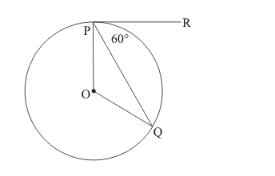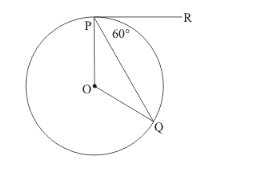# In the given figure, if PR is tangent to the circle at P and Q

Question:

In the given figure, if PR is tangent to the circle at and Q is the centre of the circle, then ∠POQ =(a) 110°

(b) 100°

(c) 120°

(d) 90°

Solution:

We know that the radius is always perpendicular to the tangent at the point of contact.Therefore, we have,

$\angle O P R=90^{\circ}$

It is given that,

$\angle Q P R=60^{\circ}$

That is,

$\angle O P R-\angle O P Q=60^{\circ}$

$90^{\circ}-\angle O P Q=60^{\circ}$

$\angle O P Q=30^{\circ}$

Now, consider $\triangle O P Q$. We have,

OP = OQ (Radii of the same circle)

Since angles opposite to equal side will be equal in a triangle, we have,We know that sum of all angles of a triangle will be equal to.

Therefore,

$\angle O P Q+\angle O Q P+\angle P O Q=180^{\circ}$

$30^{\circ}+30^{\circ}+\angle P O Q=180^{\circ}$

$\angle P O Q=120^{\circ}$

The correct answer is option (c).